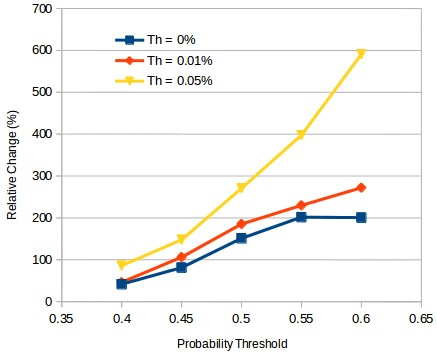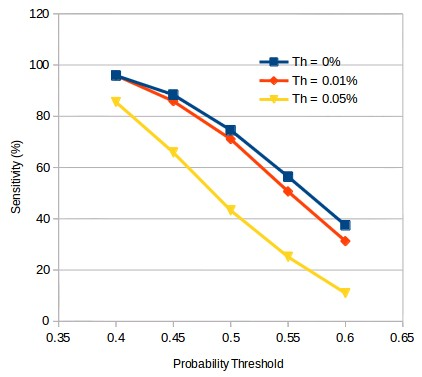## The effect of return thresholds on ML models for trading system OS predictions

On last week’s post (here) we discussed a new random forest based model that I created for the prediction of out-of-sample (OS) returns in our price-action based trading system repository at Asirikuy. This is the last from a series of currently 7 different models used for OS predictions in our community. Thinking about how I could improve these models even further I decided to implement and idea – borrowed from my work in reinforcement learning – to change the way in which the models perform classification. Today I am going to talk about what this modification is all about and how it can drastically change the results of machine learning models for OS predictions towards more positive territory. We’ll see this exemplified as I apply this modification to one of our existing random forest algorithms.When I started working in reinforcement learning for the construction of trading strategies it soon became clear that it was difficult for systems to decide when not to trade. If you always reward a system for being long or short and getting it right then the system will see that it is almost always better to either enter the market long or enter the market short. However in reality you wouldn’t want to always trade – because this level of prediction accuracy is practically impossible – but you want to only trade if you’re very certain that you will be able to make money under those market conditions. This is why it is common to introduce reward modifiers in reinforcement learning that make you only reward the trading system when it gets a long or a short right above a certain gain. That way the system won’t just always trade, but will trade only if there is a reward that’s worth it.

When building OS classifiers to determine whether or not a system should trade we can take a similar approach. Instead of trading a classifier to predict whether the system’s results will be positive or not along a given future frontier we can instead train it to predict whether the system’s average return will be above a certain threshold. The first image in this post shows you the results of doing exactly that. If we train one of our classifiers to predict the probability of a system having an average return above Th we can get a substantial improvement in the relative change of the average return within our testing set. In this case we go from a relative improvement of 2x in the average return – trading systems that are predicted to be profitable with a 60%+ probability – to a value of almost 6x when using a Th value of 0.05% instead at the same probability threshold. This means that by training our machine learning algorithm to predict the probability of a substantial profit instead of any profit, we get a significant additional gain in how we can improve our performance.However this gain does not come without consequences. As you can see in the plot above, the sensitivity of the different algorithms at the same probability threshold decreases significantly as the Th value increases. This is somewhat expected since you will be able to select less systems if you have trained the classifier for a more demanding scenario. The number of systems that will be predicted to have an average return above 0% with a 50% probability will be higher than those predicted to have an average return above 0.05% with the same probability. This is why this type of additional demand on the classification cannot be applied blindly to any algorithm, a primordial requirement is for there to be a significant sensitivity at a 50% classification threshold such that when the Th value for the algorithm is increased the sensitivity drop will not affect the selection algorithm very unfavorably.

It is also important to mention that an increase in Th is not entirely analogous to an increase in the probability threshold demand. Although a similar relative change in profitability exists for the Th|probability threshold cases 0.05%|50% and 0.01|60%, the sensitivity for the 0.05% Th algorithm at 50% is actually higher than that of the 0.01% Th algorithm at 60%. This means that the number of systems predicted to be profitable is greater for the Th 0.05% case, which means that a larger number of strategies would have traded, which is often a desirable case since higher system numbers usually imply a lower volatility in the trading result, given that the relative increase in the mean return is the same. This difference happens because the relative returns of the algorithms are not the same and some algorithms have average returns that are much smaller than other, so a higher threshold in probability is not a straight forward equivalent of a higher Th.